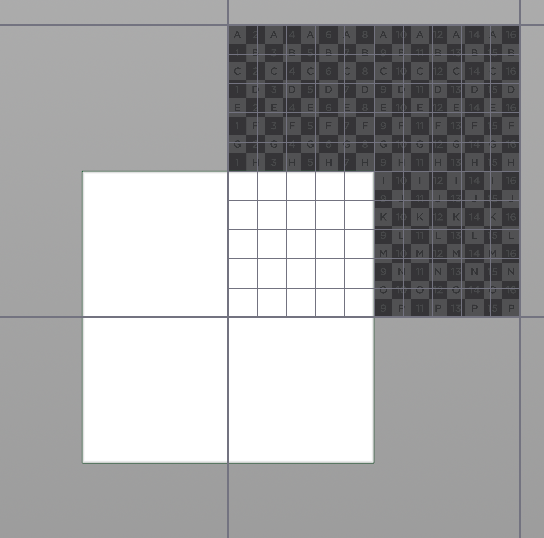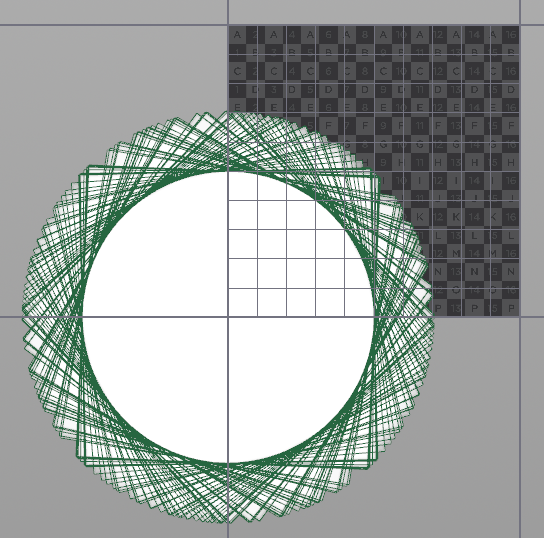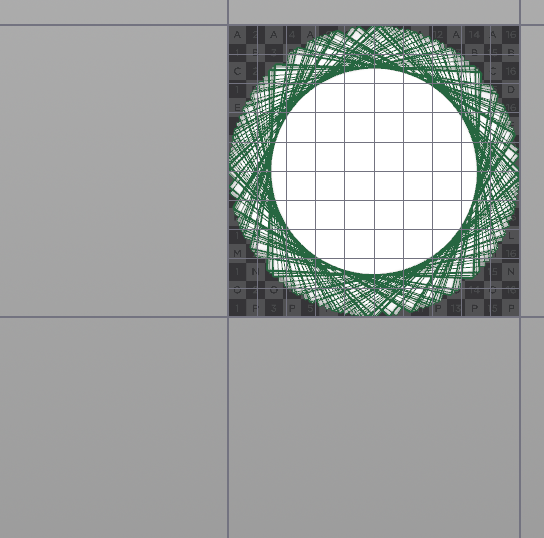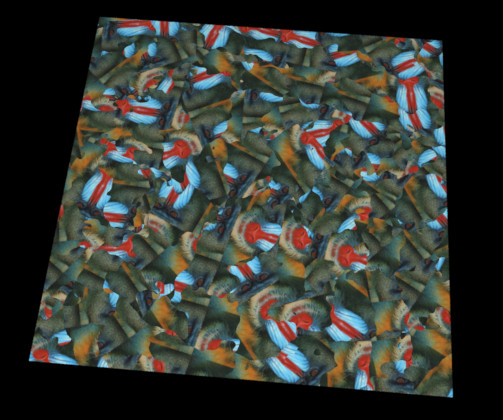## Recommended Posts

Is there a way in Houdini (VEX or VOP) to rotate each uv tiles individually, like Blender Guru does in blender ?

To break texture tiling, thanks for your help##### Share on other sites

Hi David, you can do this in:

• SOPs using primitive's node rotate and attribute copy.
• VOPs feeding random values to UV position's rotate.
• VEX feeding random values to the rotate function.
```int seed = chi('seed');

vector pivot = primuv(0, 'uv', i@primnum, 0.5);
float amount_n = rand(i@primnum, seed);
float amount_pi = fit01(amount_n, -M_PI, M_PI);

matrix3 m = ident();
rotate(m, amount_pi, {0,0,1});

v@uv = (v@uv - pivot) * m + pivot;```

rotate_UV_tiles.hipnc

•1
•1

##### Share on other sites

Or if you want overlapping UVs, using maximum space and everything in one vertex wrangle:

```string grp = itoa(i@primnum);
vector bb_prim = relbbox(0, grp, v@P).xzy;
vector bb_center = bb_prim - {0.5, 0.5, 0.0};
float amount_n = rand(i@primnum);
float amount_pi = fit01(amount_n, -M_PI, M_PI);
v@uv = bb_center;

matrix m = ident();
rotate(m, amount_pi, {0,0,1});
v@uv *= m;

float diag = sqrt(0.5);
v@uv = fit(v@uv, -diag, diag, 0.0, 1.0);```

Here are some screenshots showing what each paragraph is doing:rotate_UV_tiles_overlap.hipnc

•1

##### Share on other sites

That is really cool Konstantin thanks for that!

Can you tell if there is also a easy way to do that regardless of the surface division like in a single poly plane like this - Rotate uv tile blender tutorial

Thanks.

##### Share on other sites

Hi Michel,

yes sure. I think we have already discussed this under the keyword "texture bombing".

##### Share on other sites
1 hour ago, konstantin magnus said:

Hi Michel,

yes sure. I think we have already discussed this under the keyword "texture bombing".

Yeah I am aware of texture bombing I was hoping that someone knew a simpler way to just rotate the udims independently like Andrew does in Blender.

Thanks for the tip thou.

##### Share on other sites

I see. Here is a quick concept of a material shader which rotates a user-defined number of tiles across a UV map. It includes noise to cover up the seams a little.uv_tiles_noise.hipnc

•3

##### Share on other sites

I have tried with an heighfield, but an heighfield as too many polygons, do you know another way to randomize uv or a simple application of texture bombiing for large terrains ?

##### Share on other sites

Which method are you referring to? What's your desired output (image texture, mantra shader, vertex colors)?

##### Share on other sites
20 minutes ago, konstantin magnus said:

Which method are you referring to? What's your desired output (image texture, mantra shader, vertex colors)?

I've tried the method with overlapping uv, and I simply want to randomize the uv to break the tiling

hdni_rendermanterrain.hiplc##### Share on other sites

I guess the continuous grouping and building of bounding boxes has killed the performance. If it's quads only, you better try something like this instead:

```int vtx = vertexprimindex(0, i@vtxnum);
v@uv.x = vtx == 0 || vtx == 1;
v@uv.y = vtx == 0 || vtx == 3;
v@uv -= set(0.5, 0.5, 0.0);

float amount_n = rand(i@primnum, 123);
float amount_pi = fit01(amount_n, -M_PI, M_PI);

matrix m = ident();
rotate(m, amount_pi, {0,0,1});
v@uv *= m;

float diag = sqrt(0.5);
v@uv = fit(v@uv, -diag, diag, 0.0, 1.0);```

##### Share on other sites
Posted (edited)

Hey Konstantin,

Your help came at the right time! I'm currently trying to achieve something very similar to David's example, but I need to restrict the rotation randomness to 90 degrees.
I am familiar with some expressions to perform rotations in set increments, but not sure how to implement them with you code. I made a couple attempts but it just completely breaks your vex.

The method that seems to work best with my setup is the 4-VEX UNIT.

Much appreciated!

Edited by velk

##### Share on other sites

Hi @velk,

just cut the rotation amount values in half:

`float amount_n = rand(i@primnum) * 0.5;`

Or to get exact control over maximum degrees:

```float degrees = chf('max_degrees');

int vtx = vertexprimindex(0, i@vtxnum);
v@uv.x = vtx == 0 || vtx == 1;
v@uv.y = vtx == 0 || vtx == 3;
v@uv -= set(0.5, 0.5, 0.0);

float amount_n = rand(i@primnum, 123);

matrix m = ident();
v@uv *= m;

float diag = sqrt(0.5);
v@uv = fit(v@uv, -diag, diag, 0.0, 1.0);```

•1

##### Share on other sites
Posted (edited)

Thanks @konstantin magnus

It looks like this limits the maximum angle, however it also outputs any angle in-between.
Is there a way to strictly enforce only 0, 90, 180, 270 degrees?

I tried to adjust your random function and also played with the other methods you provided earlier (primitive, vop, vex) but I can't seem to achieve what I described.

In the Vex method from your last post, I tried variations of this:
float amount_n = (floor(rand(\$PT)*4)*90);

In the Primitive method, I tried variations of this in the rotate Y field:
floor((rand(\$PT)*2-1)*(360/90))*90

Cheers

Edited by velk

##### Share on other sites

Hi @velk, does this work for you?

```int inc = chi('steps');
float degrees = chf('max_degrees');

int vtx = vertexprimindex(0, i@vtxnum);
v@uv.x = vtx == 0 || vtx == 1;
v@uv.y = vtx == 0 || vtx == 3;
v@uv -= set(0.5, 0.5, 0.0);

float amount_n = rand(i@primnum, 123);
float amount_inc = floor(amount_n * inc) / float(inc);

matrix m = ident();
v@uv *= m;

float diag = sqrt(0.5);
v@uv = fit(v@uv, -diag, diag, 0.0, 1.0);```

##### Share on other sites
Posted (edited)

Hey @konstantin magnus

It still rotates at random orientations. See attached. I also tried with 90 and 90, and other values to test in the Max Degrees and Steps.

Is there a way to perhaps get the code to pick between a list of values (0, 90, 180, 270) instead of random?
Not the most flexible solution, but it would get the job done for simple scenarios.

Edited by velk

##### Share on other sites

No no, just set steps to 4 and max_angle to 270°.

•1

##### Share on other sites

Ahhhh got it!

With 270, it still produces irregular angles. Looks like the correct combination is Max Degree: 360 and Steps: 4.

Thanks again for your help with this!

•1

## Create an account

Register a new account